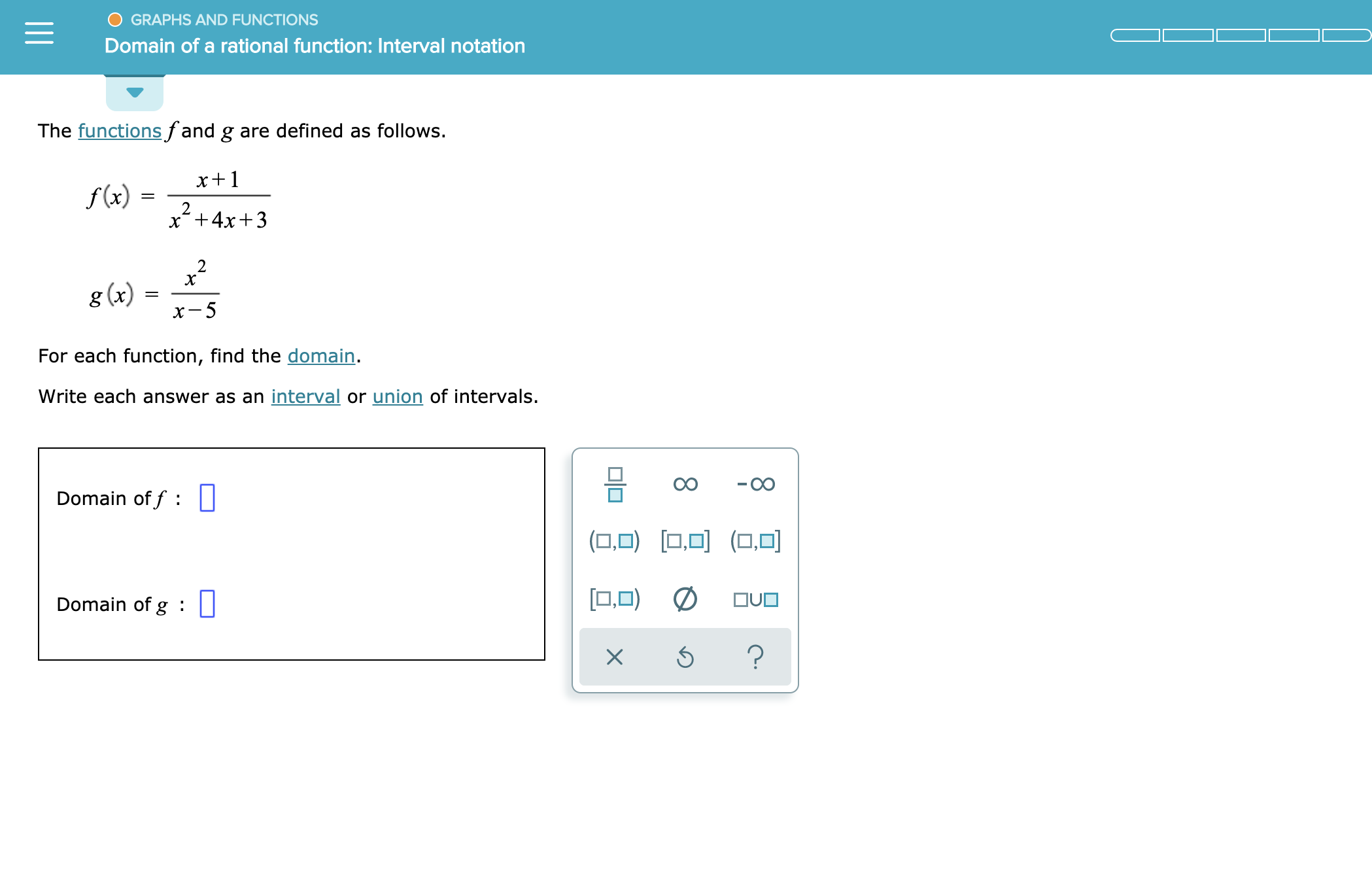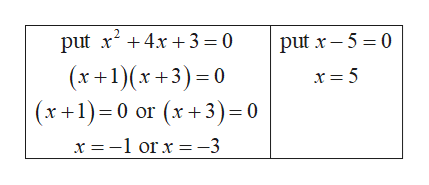# GRAPHS AND FUNCTIONSDomain of a rational function: Interval notationThe functionsfand g are defined as follows.x1f(x)==2x4x32g (x)For each function, find the domain.Write each answer as an interval or union of intervals.Domain of f : ||(O,O)OUODomain of g:?X

Question
15 views

see attachmenthelp_outlineImage TranscriptioncloseGRAPHS AND FUNCTIONS Domain of a rational function: Interval notation The functionsfand g are defined as follows. x1 f(x) == 2 x4x3 2 g (x) For each function, find the domain. Write each answer as an interval or union of intervals. Domain of f : || (O,O) OUO Domain of g: ? X fullscreen
check_circle

Step 1

The given function f(x) and g(x) are:

Step 2

To find the domain of f(x) and g(x), put denominator of f(x) and g...help_outlineImage Transcriptionclose2 put x4x 3 = 0 put x 5 0 (r+1)(x+3)0 x1)0 or x3) 0 x -1 orx = -3 fullscreen

### Want to see the full answer?

See Solution

#### Want to see this answer and more?

Solutions are written by subject experts who are available 24/7. Questions are typically answered within 1 hour.*

See Solution
*Response times may vary by subject and question.
Tagged in

### Functions